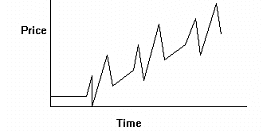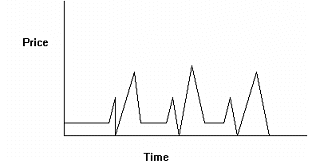# Neural Network – Output Discussion

Once you’re familiar with neural networks, you realize they can help you solve many different problems. You also know from experience there is more than one way to approach a problem. For example, if you’re creating a neural network to predict stocks, you can predict a number of things: stock price, change in a stock price, percent change in a stock price, stock volatility, etc. You could also classify the market as to whether it is going bull or bear, or you could get the network to make a buy, sell, or hold decision. In any of the above choices, you have a number of options about how far ahead you want to predict. The variables you want to predict or classify will be the neural network actual output.
Normalizing Variables (why you shouldn’t predict the Close)
Suppose you wanted to use a price indicator as a variable that has a graph over time that looks like the following graph:As you can see, a distinct pattern is evident even to our own neural networks, but this could look like three different patterns to a neural network because they occur at different price levels! Ideally, we’d like to present the data to the network as in the following graph:The way to do this is to create an indicator that normalizes the data. There are many ways this can be done, among them:

• Use price relative to some baseline, such as the last 100 days’ moving average (i.e. use the indicator X – avg(close, 100), which is found in the Simple Moving Average Category)

• Use price relative to some index which could flatten the graph

• Use change in price

• Use percent change in price

• Use ratios of two variables to make one variable whenever possible

Of course, you can avoid normalization if you don’t go so far back in time for sample patterns that the price levels were radically different.

Predict the Change (or Percent Change) of the Open vs. Close

If you are like most people, you download your data at night, run your analysis, and place your order for tomorrow’s open based upon that analysis. In this case it makes the most sense to predict the Change (or Percent Change) of the Open, not the Close. The reason for this is because the most interesting (and useful) prediction is one that predicts the difference between your entry point and your exit point (which is the open).

When you use the Change in Open or Percent Change in Open for your actual output, the NeuroShell Trader predicts the movement between the next bar’s open and the open <Trading Bars> ahead of the next bar.

Assume you could predict the Percent Change in Open for 1 trading day into the future from the next open 100% accurately. If the prediction triggered a buy signal tonight, you would know that if you bought at the open tomorrow and sold at the open the next day, your profit would be exactly what the neural net predicted.

Now assume you could predict the Percent Change in Close for 1 trading day into the future 100% accurately. If the prediction triggered a buy signal tonight, you COULD ACTUALLY LOSE MONEY if you bought at the open tomorrow and sold at the open the next day, even though your prediction is 100% accurate. You would lose money anytime the close tomorrow is higher than the close today, but the market opened higher tomorrow than it opened the day after tomorrow.

Predict the Optimal Change (or Optimal Percent Change)

The optimal change actual outputs provide a more robust interpretation of the change in price. The optimal change actual outputs compute the price movement from the current value to the next peak or valley found within the specified number of bars into the future. By doing so, they avoid the problem of premature exit signals that often occur when predicting the change.

Take as an example the case where you are in a long position and the current price is at 100. The price rises to 125 over the next 5 days and then drops down to 85 the following 5 days. If you were predicting the 10 day change in price, the change would be -15 and you would prematurely exit your position thinking the price was heading downward. In this case, you don’t want to exit the long position because there are still another 5 days and 25 points of upward price movement.

In contrast to simply predicting the change, the optimal change actual outputs only predict the price change to the next peak/valley within the specified number of bars into the future. The logic used to calculate the optimal peak/valley indicators is as follows:

1. Choose the initial peak/valley by determining if the highest price value or the lowest price value within the specified number of bars into the future will produce the largest absolute price change from the current price.
2. Continue calculating the change to the chosen peak/valley until the peak/valley is reached.
3. Once the peak or valley has been reached, start again at #1

In the example above, the optimal change indicators would initially choose the peak at 125 and continue calculating the change to 125 until 125 was reached. After that, they would then decide whether the absolute change of 40 to the valley at 85 is the next target or whether a new peak has entered the future look forward window which would have a larger absolute price change than 40 (i.e. a peak at 165 or higher).

Predicting a Peak or Valley in Prices

If you only want to predict whether you are approaching a price peak or a price valley and not the magnitude of change to the upcoming price peak or price valley, then you may want to use the Optimal Buy/Sell and the optimal Buy/Sell/Hold actual outputs. These actual outputs are calculated in the exact same manner as the optimal change outputs above, except that they provide a simple buy/sell/hold value instead of a dynamic price change.

The Optimal Buy/Sell actual output is a buy(1) when prices are going upwards towards a price peak and a sell(-1) when prices are going downwards towards a price valley.

The buy/sell/hold indicator is similar to the buy/sell except that it changes from a buy(1) to a hold(0) when the distance to the next peak is less than the distance to the valley that follows the peak. The buy/sell/hold indicator also changes from a sell(-1) to a hold(0) when the distance to the next valley is less than the distance to the peak that follows the valley.
Note that even though the Optimal Buy/Sell and Optimal Buy/Sell/Hold actual outputs are an integer value of 1/-1 or 1/0/-1, the predicted output of the neural network will still be a range of floating point numbers. The predicted output will NOT be an integer value when trying to predict the Optimal Buy/Sell and Optimal Buy/Sell/Hold.
Be Careful with Noisy Price Movement
Price indicators tend to have a lot of noisy movement in them. If you have trouble predicting price indicators, explore predicting a moving average or other indicators. If you predict a moving average, make sure that the number of bars into the future that you are predicting is at least as long as the bars you are averaging. This will insure that no component of the output is used as an input to the neural net.

Topic of Interest:
What are Neural Networks?